# 2.7 Linear inequalities and absolute value inequalities  (Page 5/11)

 Page 5 / 11

## Verbal

When solving an inequality, explain what happened from Step 1 to Step 2:

When we divide both sides by a negative it changes the sign of both sides so the sense of the inequality sign changes.

When solving an inequality, we arrive at:

$\begin{array}{l}x+2

Explain what our solution set is.

When writing our solution in interval notation, how do we represent all the real numbers?

$\left(-\infty ,\infty \right)$

When solving an inequality, we arrive at:

$\begin{array}{l}x+2>x+3\hfill \\ \phantom{\rule{1.2em}{0ex}}2>3\hfill \end{array}$

Explain what our solution set is.

Describe how to graph $\text{\hspace{0.17em}}y=|x-3|$

We start by finding the x -intercept, or where the function = 0. Once we have that point, which is $\text{\hspace{0.17em}}\left(3,0\right),$ we graph to the right the straight line graph $\text{\hspace{0.17em}}y=x-3,$ and then when we draw it to the left we plot positive y values, taking the absolute value of them.

## Algebraic

For the following exercises, solve the inequality. Write your final answer in interval notation.

$4x-7\le 9$

$3x+2\ge 7x-1$

$\left(-\infty ,\frac{3}{4}\right]$

$-2x+3>x-5$

$4\left(x+3\right)\ge 2x-1$

$\left[\frac{-13}{2},\infty \right)$

$-\frac{1}{2}x\le \frac{-5}{4}+\frac{2}{5}x$

$-5\left(x-1\right)+3>3x-4-4x$

$\left(-\infty ,3\right)$

$-3\left(2x+1\right)>-2\left(x+4\right)$

$\frac{x+3}{8}-\frac{x+5}{5}\ge \frac{3}{10}$

$\left(-\infty ,-\frac{37}{3}\right]$

$\frac{x-1}{3}+\frac{x+2}{5}\le \frac{3}{5}$

For the following exercises, solve the inequality involving absolute value. Write your final answer in interval notation.

$|x+9|\ge -6$

All real numbers $\text{\hspace{0.17em}}\left(-\infty ,\infty \right)$

$|2x+3|<7$

$|3x-1|>11$

$\left(-\infty ,\frac{-10}{3}\right)\cup \left(4,\infty \right)$

$|2x+1|+1\le 6$

$|x-2|+4\ge 10$

$\left(-\infty ,-4\right]\cup \left[8,+\infty \right)$

$|-2x+7|\le 13$

$|x-7|<-4$

No solution

$|x-20|>-1$

$|\frac{x-3}{4}|<2$

$\left(-5,11\right)$

For the following exercises, describe all the x -values within or including a distance of the given values.

Distance of 5 units from the number 7

Distance of 3 units from the number 9

$\left[6,12\right]$

Distance of10 units from the number 4

Distance of 11 units from the number 1

$\left[-10,12\right]$

$-4<3x+2\le 18$

$3x+1>2x-5>x-7$

$3y<5-2y<7+y$

$x+7

## Graphical

For the following exercises, graph the function. Observe the points of intersection and shade the x -axis representing the solution set to the inequality. Show your graph and write your final answer in interval notation.

$|x-1|>2$

$\left(-\infty ,-1\right)\cup \left(3,\infty \right)$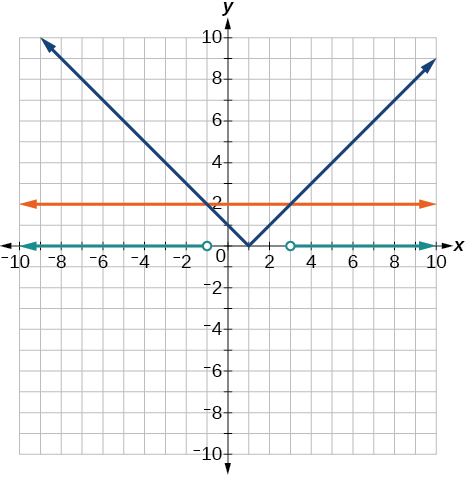$|x+3|\ge 5$

$|x+7|\le 4$

$\left[-11,-3\right]$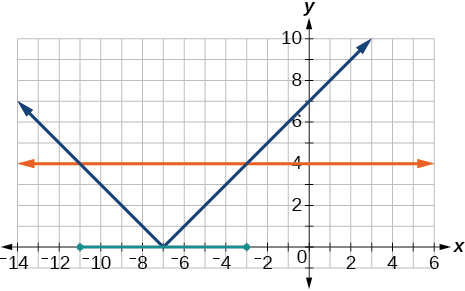$|x-2|<7$

$|x-2|<0$

It is never less than zero. No solution.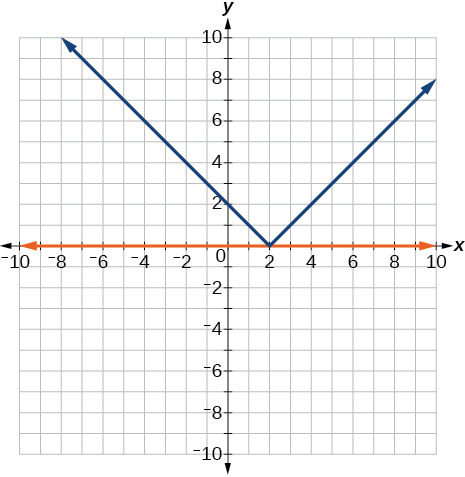For the following exercises, graph both straight lines (left-hand side being y1 and right-hand side being y2) on the same axes. Find the point of intersection and solve the inequality by observing where it is true comparing the y -values of the lines.

$x+3<3x-4$

$x-2>2x+1$

Where the blue line is above the orange line; point of intersection is $\text{\hspace{0.17em}}x=-3.$

$\left(-\infty ,-3\right)$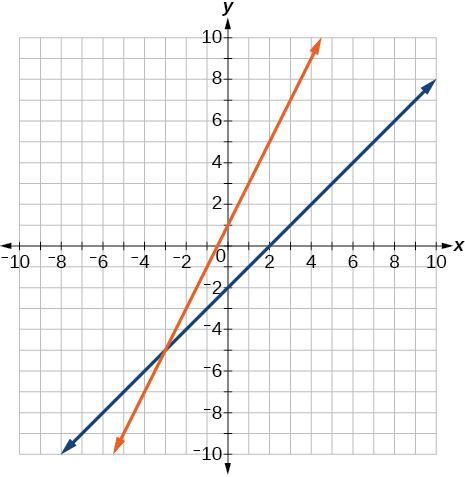$x+1>x+4$

$\frac{1}{2}x+1>\frac{1}{2}x-5$

Where the blue line is above the orange line; always. All real numbers.

$\left(-\infty ,-\infty \right)$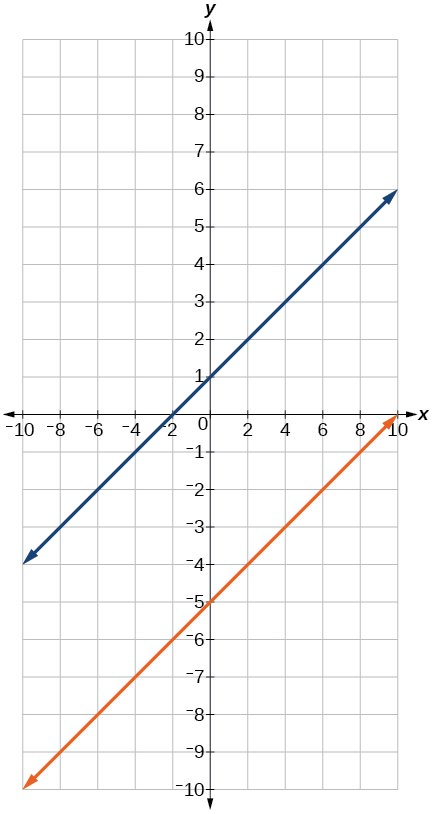$4x+1<\frac{1}{2}x+3$

## Numeric

For the following exercises, write the set in interval notation.

$\left\{x|-1

$\left(-1,3\right)$

$\left\{x|x\ge 7\right\}$

$\left\{x|x<4\right\}$

$\left(-\infty ,4\right)$

For the following exercises, write the interval in set-builder notation.

$\left(-\infty ,6\right)$

$\left\{x|x<6\right\}$

$\left(4,+\infty \right)$

$\left[-3,5\right)$

$\left\{x|-3\le x<5\right\}$

$\left[-4,1\right]\cup \left[9,\infty \right)$

For the following exercises, write the set of numbers represented on the number line in interval notation.

use the y -intercept and slope to sketch the graph of the equation y=6x
how do we prove the quadratic formular
hello, if you have a question about Algebra 2. I may be able to help. I am an Algebra 2 Teacher
thank you help me with how to prove the quadratic equation
Seidu
may God blessed u for that. Please I want u to help me in sets.
Opoku
what is math number
4
Trista
x-2y+3z=-3 2x-y+z=7 -x+3y-z=6
Need help solving this problem (2/7)^-2
x+2y-z=7
Sidiki
what is the coefficient of -4×
-1
Shedrak
the operation * is x * y =x + y/ 1+(x × y) show if the operation is commutative if x × y is not equal to -1
An investment account was opened with an initial deposit of \$9,600 and earns 7.4% interest, compounded continuously. How much will the account be worth after 15 years?
lim x to infinity e^1-e^-1/log(1+x)
given eccentricity and a point find the equiation
12, 17, 22.... 25th term
12, 17, 22.... 25th term
Akash
College algebra is really hard?
Absolutely, for me. My problems with math started in First grade...involving a nun Sister Anastasia, bad vision, talking & getting expelled from Catholic school. When it comes to math I just can't focus and all I can hear is our family silverware banging and clanging on the pink Formica table.
Carole
I'm 13 and I understand it great
AJ
I am 1 year old but I can do it! 1+1=2 proof very hard for me though.
Atone
Not really they are just easy concepts which can be understood if you have great basics. I am 14 I understood them easily.
Vedant
hi vedant can u help me with some assignments
Solomon
find the 15th term of the geometric sequince whose first is 18 and last term of 387
I know this work
salma
The given of f(x=x-2. then what is the value of this f(3) 5f(x+1)
hmm well what is the answer
Abhi
If f(x) = x-2 then, f(3) when 5f(x+1) 5((3-2)+1) 5(1+1) 5(2) 10
AugustineByByByBy Eric Crawford